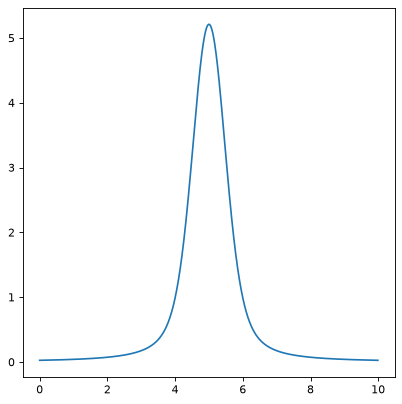# Voigt1D¶

class astropy.modeling.functional_models.Voigt1D(x_0=0, amplitude_L=1, fwhm_L=0.6366197723675814, fwhm_G=0.6931471805599453, **kwargs)[source]

One dimensional model for the Voigt profile.

Parameters: Other Parameters: x_0 : float Position of the peak amplitude_L : float The Lorentzian amplitude fwhm_L : float The Lorentzian full width at half maximum fwhm_G : float The Gaussian full width at half maximum fixed : a dict, optional A dictionary {parameter_name: boolean} of parameters to not be varied during fitting. True means the parameter is held fixed. Alternatively the fixed property of a parameter may be used. tied : dict, optional A dictionary {parameter_name: callable} of parameters which are linked to some other parameter. The dictionary values are callables providing the linking relationship. Alternatively the tied property of a parameter may be used. bounds : dict, optional A dictionary {parameter_name: value} of lower and upper bounds of parameters. Keys are parameter names. Values are a list or a tuple of length 2 giving the desired range for the parameter. Alternatively, the min and max properties of a parameter may be used. eqcons : list, optional A list of functions of length n such that eqcons[j](x0,*args) == 0.0 in a successfully optimized problem. ineqcons : list, optional A list of functions of length n such that ieqcons[j](x0,*args) >= 0.0 is a successfully optimized problem.

Notes

Algorithm for the computation taken from McLean, A. B., Mitchell, C. E. J. & Swanston, D. M. Implementation of an efficient analytical approximation to the Voigt function for photoemission lineshape analysis. Journal of Electron Spectroscopy and Related Phenomena 69, 125-132 (1994)

Examples

import numpy as np
from astropy.modeling.models import Voigt1D
import matplotlib.pyplot as plt

plt.figure()
x = np.arange(0, 10, 0.01)
v1 = Voigt1D(x_0=5, amplitude_L=10, fwhm_L=0.5, fwhm_G=0.9)
plt.plot(x, v1(x))
plt.show()


()Attributes Summary

 amplitude_L fwhm_G fwhm_L input_units This property is used to indicate what units or sets of units the evaluate method expects, and returns a dictionary mapping inputs to units (or None if any units are accepted). param_names x_0

Methods Summary

 evaluate(x, x_0, amplitude_L, fwhm_L, fwhm_G) Evaluate the model on some input variables. fit_deriv(x, x_0, amplitude_L, fwhm_L, fwhm_G)

Attributes Documentation

amplitude_L = Parameter('amplitude_L', value=1.0)
fwhm_G = Parameter('fwhm_G', value=0.6931471805599453)
fwhm_L = Parameter('fwhm_L', value=0.6366197723675814)
input_units

This property is used to indicate what units or sets of units the evaluate method expects, and returns a dictionary mapping inputs to units (or None if any units are accepted).

Model sub-classes can also use function annotations in evaluate to indicate valid input units, in which case this property should not be overridden since it will return the input units based on the annotations.

param_names = ('x_0', 'amplitude_L', 'fwhm_L', 'fwhm_G')
x_0 = Parameter('x_0', value=0.0)

Methods Documentation

classmethod evaluate(x, x_0, amplitude_L, fwhm_L, fwhm_G)[source]

Evaluate the model on some input variables.

classmethod fit_deriv(x, x_0, amplitude_L, fwhm_L, fwhm_G)[source]